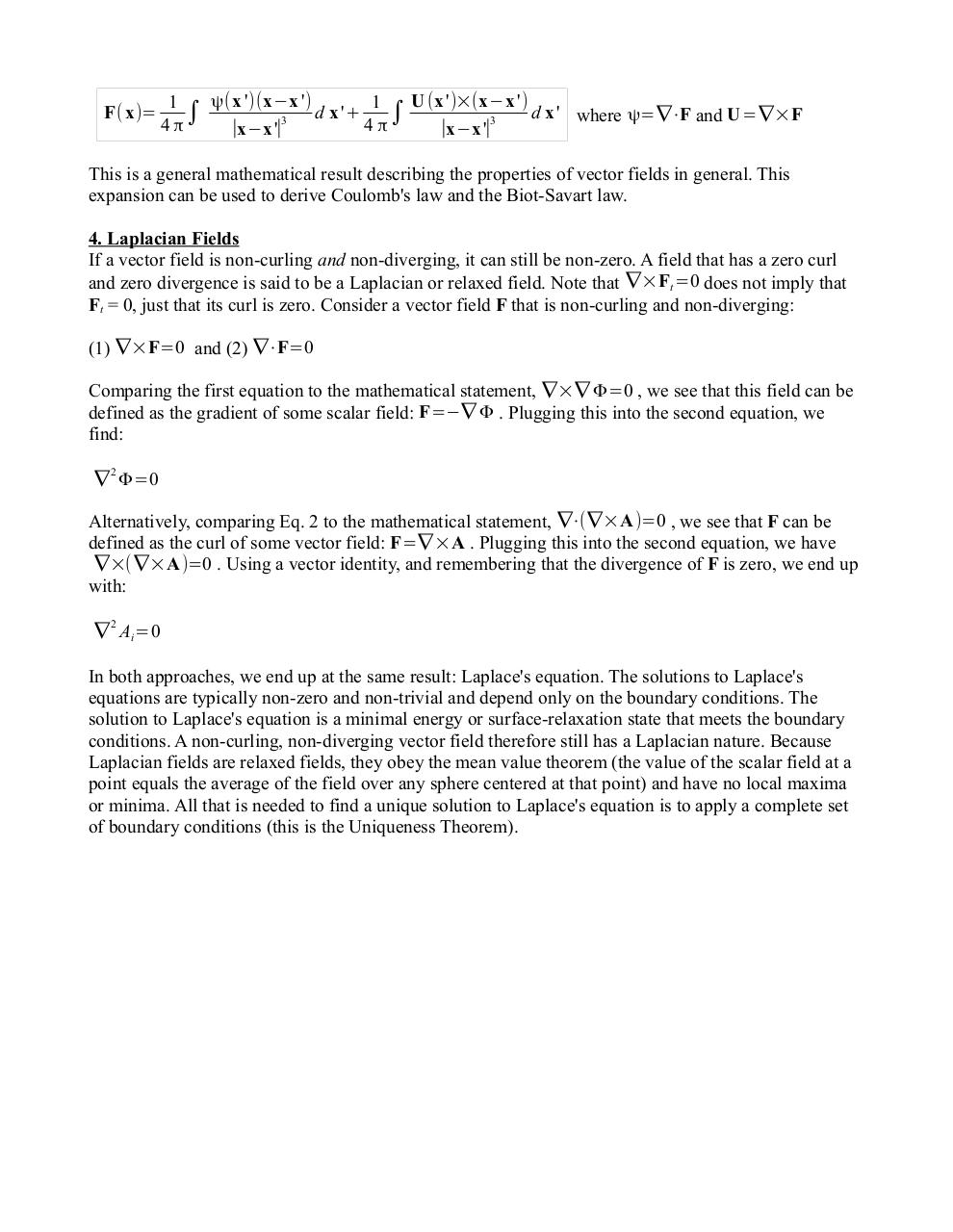# SuppHelmholtzDecomposition.pdfPage 1 2 3 4 5 6

#### Text preview

F( x)=

ψ( x ')(x−x ')
U (x ')×(x−x ')
1
1
d x '+
d x ' where ψ=∇⋅F and U=∇×F

3

∣x−x '∣
∣x−x '∣3

This is a general mathematical result describing the properties of vector fields in general. This
expansion can be used to derive Coulomb's law and the Biot-Savart law.
4. Laplacian Fields
If a vector field is non-curling and non-diverging, it can still be non-zero. A field that has a zero curl
and zero divergence is said to be a Laplacian or relaxed field. Note that ∇×Ft =0 does not imply that
Ft = 0, just that its curl is zero. Consider a vector field F that is non-curling and non-diverging:
(1) ∇×F=0 and (2) ∇⋅F=0
Comparing the first equation to the mathematical statement, ∇×∇ Φ=0 , we see that this field can be
defined as the gradient of some scalar field: F=−∇ Φ . Plugging this into the second equation, we
find:
2

∇ Φ=0

Alternatively, comparing Eq. 2 to the mathematical statement, ∇⋅(∇×A)=0 , we see that F can be
defined as the curl of some vector field: F=∇ ×A . Plugging this into the second equation, we have
∇×(∇×A)=0 . Using a vector identity, and remembering that the divergence of F is zero, we end up
with:
∇ 2 Ai=0
In both approaches, we end up at the same result: Laplace's equation. The solutions to Laplace's
equations are typically non-zero and non-trivial and depend only on the boundary conditions. The
solution to Laplace's equation is a minimal energy or surface-relaxation state that meets the boundary
conditions. A non-curling, non-diverging vector field therefore still has a Laplacian nature. Because
Laplacian fields are relaxed fields, they obey the mean value theorem (the value of the scalar field at a
point equals the average of the field over any sphere centered at that point) and have no local maxima
or minima. All that is needed to find a unique solution to Laplace's equation is to apply a complete set
of boundary conditions (this is the Uniqueness Theorem).